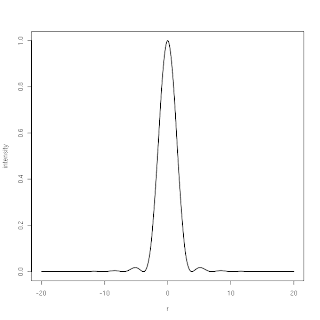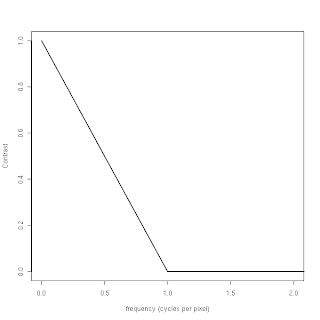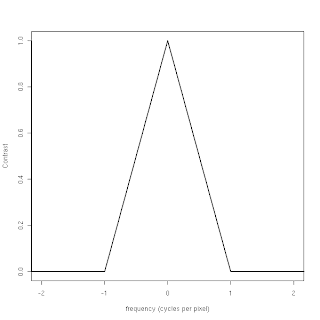## Wednesday, 6 June 2012

### Incorporating diffraction

A while ago I posted an article on the MTF of a sensor without an AA filter in the absence of diffraction (here if you've missed it). By not including the effects of diffraction, we could see how the lack of an AA filter produced significant aliasing, which shows up visually as false detail.

Now it is time to add the effects of diffraction back in. I do not have the required background in physics to explain this from first principles, but we can probably blame diffraction on the wave properties of light. If we take a beam of light and pass it through a narrow vertical slit, and then project it onto a surface, we do not see quite what we expected.

we actually see thisIntensity pattern of diffraction through narrow slit

Of course, to illustrate this principle I had to compress the intensity of the diffraction image quite a bit, since the secondary stripes are much dimmer than the main one. Making the slit narrower causes the intensity pattern to spread out more.
The intensity pattern that we observe is in fact sinc2(x), and looks like this:Intensity profile of narrow slit, sinc2(x)
Recall that we first observed the sinc(x) function, which is defined as
sinc(x) = sin(x) / (x),
when we computed the Fourier transform of the box function. Note that a cross-section of the vertical slit (along constant y values) is in fact a box function. Why is the intensity function sinc2(x) rather than sinc(x) ? Again, I lack the physics background to explain this properly, but it is squared because we are dealing with incoherent light, which is a safe assumption for typical photographic light sources. Coherent light sources, such as lasers, will have a sinc(x) diffraction pattern upon passing through a narrow slit.
If we have a square hole (aperture) rather than a vertical slit, we will observe this intensity pattern:Intensity pattern of square aperture

which is simply sinc2(x)*sinc2(y).

If we want to introduce a circular aperture, like the one in a real lens, then we can no longer treat x and y separately. A circular aperture produces an intensity pattern that looks like this:Intensity pattern of circular aperture (Airy pattern)
This pattern is also known as the Airy pattern, with the bright central circular area (before first black ring) known as the Airy disc. Again, a smaller aperture causes the size of this disc to increase, and the entire pattern to spread out.

Although x and y can no longer be treated independently, we can transform them to polar coordinates to obtain r = sqrt(x2 + y2), which yields the intensity function jinc2(r) where
jinc(r) = 2*BesselJ(r)/(r)
and BesselJ is a Bessel function of the first kind, of order 1 (see Wikipedia). Since this function is radially symmetrical (as can be seen in the intensity image above) we can plot it simply as a function of rIntensity profile (radial) of circular aperture, jinc2(r)

The result is that the circular aperture behaves somewhat like a square aperture, except that instead of observing a sinc2(x) function, we instead observe a jinc2(r). Note that the side-lobes of the jinc2(r) function are much smaller than those of the sinc2(x) --- you can probably guess what effect this will have on MTF.

### Diffraction MTF

So what does the MTF curve of a diffraction pattern look like? For the first case, namely the MTF of the diffraction pattern of a vertical slit, we can perform the analysis in one dimension, to obtain the following curve:MTF of sinc2(x) PSF

If you have guessed that it looks an awful lot like a straight line, then you guessed correctly. Recall that the intensity function of the vertical slit is sinc2(x). The Fourier transform of sinc(x) is a box function (see here for a recap), and vice versa, so the MTF of sinc(x) is just a box function in the frequency domain. Since multiplication in the time (or spatial) domain is equivalent to convolution in the frequency domain, we can see that the Fourier transform of sinc2(x) must be the convolution of a box function with itself in the frequency domain. Lastly, recall that the convolution of a box filter with itself (once only) yields a triangle function, and we are only plotting the positive frequencies in the MTF plot, i.e., you can mirror the MTF around the y axis to see the triangle more clearly, like this:MTF of sinc2(x) PSF, showing negative and positive frequencies

This result extends to the MTF of the diffraction pattern of a square aperture, since x and y can simply be treated independently. As you have probably guessed, the 2D MTF of the square aperture looks like this:MTF (left) and PSF (right) of square aperture
Note that this MTF shape can also be obtained through the convolution of two 2D box functions.

You may be tempted to treat the circular aperture in the same way, but trying to compute the MTF in one dimension will not give you the correct result. If you can visualise the MTF of the square aperture as the convolution of a box function with itself, then you can visualise the MTF of the circular aperture as the convolution of two "pillbox" functions that look like this:A pillbox function
The convolution of two of these pillbox functions will give you the MTF on the left:MTF (left) and PSF (right) of circular aperture
which is called the Chinese hat function, or chat(x). We can take a cross-section through the centre of this 2D function to obtain this plot:chat(f) = MTF of jinc2(r)
Note that this function is very nearly a triangle function --- except that it curves a bit. This should not be totally surprising, since you can imagine that you could fit a square box function inscribed in the cylinder formed by the pillbox function.

If we focus only on the positive half of the frequency spectrum, the function can be expressed as
chat(s) = 2/π * (acos(s) - s*√(1 - s2))
which is the form that you will usually see in optics literature. Now we know what the MTF curve looks like, so we can obtain the corresponding point spread function by computing the inverse Fourier transform of the MTF curve.

If you perform this inversion in two dimensions, and then take a cross-section through the result, you will obtain the known intensity function, jinc2(r).
Right. Now sample the jinc2(r) function in one dimension, and apply the Fast Fourier Transform (FFT) to obtain the 1D MTF:Trying to obtain chat(f) by computing FFT of jinc2(r)
Whoops! That does not work as expected. You have to perform the calculations in 2D for this to work. It only took me a (tortuous) weekend to discover this useful fact. If only someone had warned me ...

### Other useful facts regarding diffraction

Just as with a Gaussian, the width of the MTF function is inversely proportional to the width of the PSF, i.e., smaller apertures produce larger diffraction patterns.

Diffraction is wavelength-dependent. If jinc2(r) is your PSF, then r should be expressed as
r = π * d / (λ * N)
where d is your radial distance from the optical axis, as measured in the focal plane. If you are modelling the diffraction PSF in terms of pixels, then you can express d in pixels, but you must adjust λ accordingly, such that
rp = π * dp / ( (λ/Δ) * N)
where Δ denotes your pixel pitch, expressed in the same units as λ. For example, the Nikon D800 has a pixel pitch of 4.88 micron, and green light has a wavelength of 550 nm, or 0.55 micron. This means that the diffraction PSF expressed in D800 pixel-units would be
rpπ * dp / ( 0.112705 * N).

If we want to obtain the equivalent function in the frequency domain, i.e., the diffraction MTF, we use the scale
sp = (λ/Δ) * N * fp
where fp  is the frequency scale in cycles per pixel, and sp is normalized frequency we plug into the equation
chat(sp) = 2/π * (acos(sp) - sp*√(1 - sp2)).
For the D800 pixel size, we would use
sp ≈ 0.112705 * N * fp

In a future post, some examples of MTF curves, incorporating diffraction, will be investigated using the D800E as example.